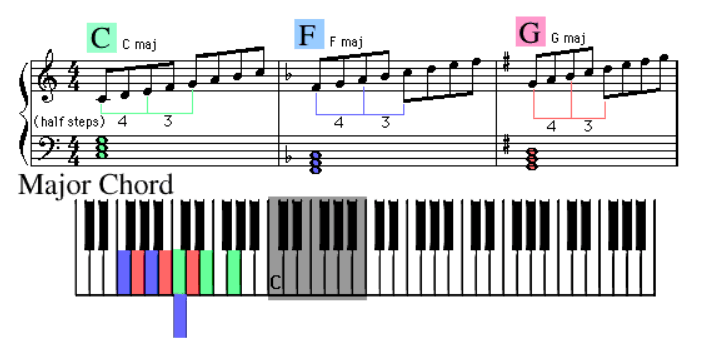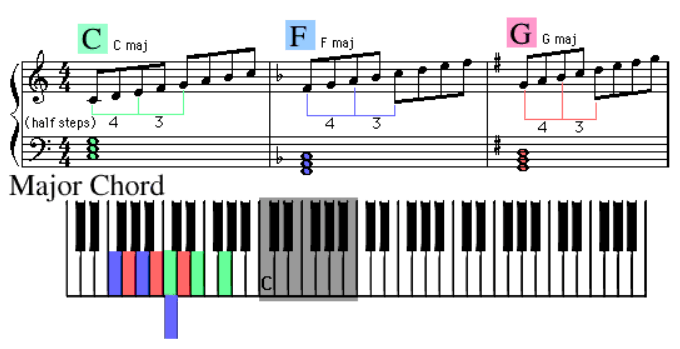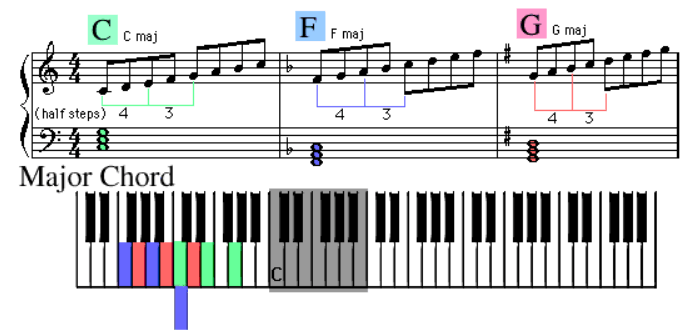html web templatesIntermediate Studies

Lesson 8 - Section 1

Chord Study

Let’s look at the chords used in the “Balloon” song from our earlier lessons. Please refer to the image for this section. The notes of the chords are written in the Bass Clef of the staff. The chords are also written above the Treble Clef in text format:

C or C maj
F or F maj
G or G maj

As you can see from the color coded notes on the image of the keyboard, the formula for constructing a Major chord is:

4 half steps + 3 half steps

Play the C Major chord (green notes) on your keyboard. Now play the F Major chord and then the G Major chord. Major chords are this easy. In fact, almost all chords are this easy if you know the formula to construct them.

Let’s look at some other formulas:

Minor chords (such as Am, Dm, and Em)
3 half steps + 4 half steps

Dominant (flatted) seventh chords (such as C7, F7, and G7)
4 half steps + 3 half steps + 3 half steps

Minor seventh chords (such as Am7, Dm7, and Em7)
3 half steps + 4 half steps + 3 half steps

Major sixth chords (such as C6, F6, and G6)
4 half steps + 3 half steps + 2 half steps

Major seventh chords (such as Cmaj7, Fmaj7, and Gmaj7)
4 half steps + 3 half steps + 4 half steps

Play each one of these types of chords on your keyboard. Use the formulas shown to construct each chord. Do this 3 different times using the root notes C, F, and G.

Now try building some Major chords on sharps and flats. Use the root notes E flat, A flat, C sharp and F sharp.

Spend about 20 minutes doing this.Lesson 8 - Section 2

Simplified by Using Thirds

Next, let’s begin to simplify the chord formulas using whole and half steps.

The formula for constructing a Major chord would be:

Two whole steps + one and one half whole steps

Using Major and Minor Thirds to Build Chords

Two whole steps is called a Major third. Any note combination that is two whole steps apart anywhere on the keyboard is called a Major third

One and one half whole steps is called a minor third. Any note combination that is one and one half whole steps apart anywhere on the keyboard is called a minor third

Now, using major and minor thirds, let’s construct our finished formulas:

Minor chords (such as Am, Dm, and Em)
minor third + major third

Dominant seventh chords (such as C7, F7, and G7)
major third + minor third + minor third

Minor seventh chords (such as Am7, Dm7, and Em7)
minor third + major third + minor third

Major sixth chords (such as C6, F6, and G6)
major third + minor third + 1 whole step (see note*)

Major seventh chords (such as Cmaj7, Fmaj7, and Gmaj7)
major third + minor third + major third

* Note: one whole step is called a Major second. Any note combination that is one whole step apart anywhere on the keyboard is called a Major second

Record all of these chord formulas in your music note book.

You can also note that:

Major third = 4 half steps
minor third = 3 half steps
Major second = 2 half steps
minor second = 1 half stepLesson 8 - Section 3

Chord Construction

Refer to the image for this section.

Consider that Major sixth chords such as C6, F6, and G6 would use the number VI (6th) note in each scale.

Consider Major seventh chords such as Cmaj7, Fmaj7, and Gmaj7 would use the number VII (7th) note in each scale.

Question: If you saw the chords C9, F9, and G9, which top note in the chord would you expect to play?

Answer: 9 notes away from the root note.

For a C9 chord that would be the root plus 9 notes, which would be a D note

For an F9 chord that would be the root plus 9 notes, which would be a G note

For a G9 chord that would be the root plus 9 notes, which would be an A note

Starting to make sense yet?

When you see a chord name like C6, it is telling you to play the chord C Major and also add the number VI (6th) note.

Congratulations! You now have the method and the knowledge to figure out any chord written for the piano! There are a few more tricks to learn but with what you’ve learned today, you’ll be able to figure out about 85% of all the chords you’ll ever see that are written using the chords letters and numbers such as D6.

Chords are like the different scales (with different numbers of sharps or flats). The more you use them, the more you will remember them without having to go through the construction process each time.

Remember . . . chords are just a group of notes that like to hang out together and help a melody line along the way.